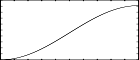## GEN19

GEN19 — Generate composite waveforms made up of weighted sums of simple sinusoids.

## Description

These subroutines generate composite waveforms made up of weighted sums of simple sinusoids. The specification of each contributing partial requires 4 p-fields using GEN19.

## Syntax

`f # time size  19  pna   stra  phsa  dcoa  pnb strb  phsb  dcob  ...`

## Initialization

size -- number of points in the table. Must be a power of 2 or power-of-2 plus 1 (see f statement).

pna, pnb, etc. -- partial no. (relative to a fundamental that would occupy size locations per cycle) of sinusoid a, sinusoid b, etc. Must be positive, but need not be a whole number, i.e., non-harmonic partials are permitted. Partials may be in any order.

stra, strb, etc. -- strength of partials pna, pnb, etc. These are relative strengths, since the composite waveform may be rescaled later. Negative values are permitted and imply a 180 degree phase shift.

phsa, phsb, etc. -- initial phase of partials pna, pnb, etc., expressed in degrees.

dcoa, dcob, etc. -- DC offset of partials pna, pnb, etc. This is applied after strength scaling, i.e. a value of 2 will lift a 2-strength sinusoid from range [-2,2] to range [0,4] (before later rescaling).

NoteThese subroutines generate stored functions as sums of sinusoids of different frequencies. The two major restrictions on GEN10 that the partials be harmonic and in phase do not apply to GEN09 or GEN19. In each case the composite wave, once drawn, is then rescaled to unity if p4 was positive. A negative p4 will cause rescaling to be skipped.

## Examples

Here is an example of the GEN19 routine. It uses the files gen19.csd.

Example 1294. An example of the GEN19 routine.

```<CsoundSynthesizer>
<CsOptions>
; Select audio/midi flags here according to platform
-odac      ;;;realtime audio out
; For Non-realtime ouput leave only the line below:
; -o gen19.wav -W ;;; for file output any platform
</CsOptions>
<CsInstruments>

sr = 44100
ksmps = 32
nchnls = 2
0dbfs  = 1

instr 1

;p4 = transposition factor
;p5 = speed factor
;p6 = function table for grain data
i1    =	sr/ftlen(1)							;scaling to reflect sample rate and table length
a1    phasor i1*p5							;index for speed
asigl fog    .5, 15, p4, a1, 1, 0, .01, .5, .01, 30, 1, 2, p3 		;left channel
asigr fog    .4, 25, p4+.2, a1, 1, 0, .01, .5, .01, 30, 1, 2, p3, .5	;right channel
outs   asigl, asigr
endin

</CsInstruments>
<CsScore>
f 1 0 131072 1 "fox.wav" 0 0 0
f 2 0 1024 19 .5 .5 270 .5

i 1 0 10 .7  .1
i 1 + 4  1.2  2
e
</CsScore>
</CsoundSynthesizer>
```

This is the diagram of the waveform of the GEN19 routines, as used in the example:f 2 0 1024 19 .5 .5 270 .5 - a rising sigmoid